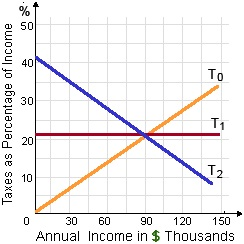When people purchase goods

People will purchase goods when their demand prices equivalent or surpass: (i) Transaction costs. (ii) Subjective prices. (iii) Price indexes. (iv) Market prices. (v) Wholesale prices.

#### Related Questions in Macroeconomics

• ##### Q :Merger and acquisition of firms

Question:

Suppose firm 1 and firm 2 merge. Call the new firm A. It has output xA and profit πA. Suppose there is Cournot competition after the merger. For now, we assume that the marginal cost of Firm A, the mer

• ##### Q :What is Bank rate Bank rate : This is

Bank rate: This is the rate at which the central bank loans money to commercial bank.

• ##### Q :Unemployment (a) Do you think that

(a) Do you think that macroeconomic policy should be designed to achieve a measured unemployment rate of zero?

• ##### Q :Important issues in Macroeconomics to

Macroeconomics is primarily focused on issues about: (w) economy extensive aggregate variables as like national income. (x) the structure of economic activity quite than its level. (y) resource allocations through households and business firms. (z) po

• ##### Q :Value of MPC when MPS is zero Determine

Determine the value of MPC whenever MPS is zero?

Answer: Whenever MPS = 0, MPC = 1 – 0 = 1.

• ##### Q :Tax system problem In the figure shown

In the figure shown below, line T1 depicts a tax system which is: (1) Regressive. (2) Progressive.  (3) Proportional. (4) Unbiased. (5) Recessive.• ##### Q :International trade the most frequently

the most frequently asked question on foreign direct invetment

• ##### Q :Demand according to range of adjustments

As longer time periods are taken and a bigger range of adjustments (or substitutions) become obtainable, then demand curves tend to become: (1) flatter, as supply curves become steeper. (2) Steeper as supply curves become flatter. (3) Flatter, and therefore do supply

• ##### Q :National income how to calculate

how to calculate national income under value added method

• ##### Q :Weighed marginal cost and marginal

Cite examples of recent decisions that you made in which you, at least implicitly, weighed marginal cost and marginal benefit?